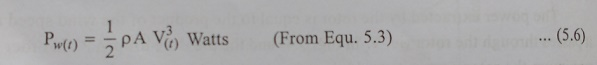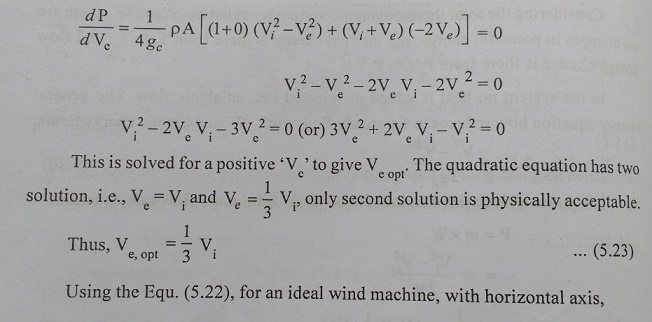Home | | Electric Energy Generation, Utilization and Conservation | Basic Principles of Wind Energy Conversion

# Basic Principles of Wind Energy Conversion

The circulation of air in the atmosphere is caused by the non-uniform heating of the earth’s surface by the sun. The air immediately above a warm area expands, it is forced upward by cool, denser air which flows in from surrounding areas causing wind.

BASIC PRINCIPLES OF WIND ENERGY CONVERSION

## 1. The Nature of Wind

The circulation of air in the atmosphere is caused by the non-uniform heating of the earth’s surface by the sun. The air immediately above a warm area expands, it is forced upward by cool, denser air which flows in from surrounding areas causing wind.

The nature of the terrain, the degree of cloud and the angle of the sun in the sky are all factors which influences this process.

In general, during the day the air above the land mass tends to heat up more rapidly than the air over water. In coastal regions this manifests itself in a strong onshore wind. At night the process is reversed because the air cools down more rapidly over the land and the breeze therefore blows off shore.

Despite the wind’s intermittent nature, wind patterns at any particular site remain remarkably constant year by year. Average wind speeds are greater in hilly and costal area than they are well inland. The winds also tend to blow more consistently and with greater strength over the surface of the water where there is a less surface drag.

## 2. The Power in Wind

Wind possesses energy by virtue of its motion. Any device capable of slowing down the mass of moving air, like a sail or propeller, can extract part of the energy and convert is into useful work.

There are three factors determine the output power generated from the wind mill, they are

(1)   The wind speed

(2)   The cross section of wind swept by rotor, and

(3)   The overall conversion efficiency of rotor, transmission system and generator or pump.

No device, however well-designed, can extract all of the wind’s energy because the wind would have to be brought to a halt and this would prevent the passage of more air through the rotor. The most that is possible is for the rotor to decelerate to whole horizontal column of intercepted air to about one-third of its free velocity.

A 100% efficient aerogenerator would therefore only be able to convert up to a maximum of around 60% of the available energy in wind into mechanical energy.

A well-designed blades will typically extract 70% of the theoretical maximum, but losses incurred in the gear box, transmission system and generator or pump could decrease overall wind tubine efficiency to 35% or less.

### 2.1 Calculation of Power in the Wind

The power in the wind can be computed by using of Kinetics (Kinetic means relating to or resulting from motion). The wind mill works on the principle of converting Kinetic energy of the wind to mechanical energy.

We know that power is equal to energy per unit time. The energy available is the kinetic energy of the wind. The kinetic energy of any particle is equal to one half its mass times the square of its velocity.Kinetic Energy of particle = ½ mv2

Where

M : Mass of particle (kg)

V : Velocity of particle (m/s)

The amount of air passing in unit time, through an area ‘A’, with velocity ‘V’ is A x V, and its mass ‘m’ is equal to its volume multiplied by its density ‘ρ’ of air.m = ρ AV

Where, m is the mass of air transversing the area ‘A’ swept by the rotating blades of a wind mill type generator.### 2.2 Power Coefficient

The fraction of the free-flow wind power that can be extracted by the rotor is called the power co-efficient; Thus,

Power Coefficient = Power of wind rotor / Power available in the wind

Where, power available is calculated from the air density, rotor diameter and free wind speed as discussed earlier. The maximum theoretical power coefficient is equal to 16/27 or 0.593. This value cannot be exceeded by a rotor in free-flow wind-stream.

An ideal rotor, with propeller-type blades of proper aerodynamic design, would have a power co-efficient approaching 0.59. But such a rotor would not be strong enough to withstand the stresses to which it is subjected when rotating at a high rate in a high-speed wind stream.

### 2.3 Instantaneous Wind Power

‘V’, in actuality, is not constant but is represented by a statically ‘Noisy’ wind speed time curve, V(t) then the instantaneous power, in the wind would be,Since, we are normally more interested in average power, we must take time average of both sides o Equ. (5.6), signified by the bar, and written as,Equ. (5.7) tells us that for a non-steady state wind, it is necessary to cube the measured wind speeds and take the average to find the average wind power available.

It is immediately obvious that this non-steady state case is more complex than the simple steady state case, and it is why for the former case such great emphasis is placed on anemometry data at a proposed Wind Energy Conversion System (WECS) site.

Transposing Equ. (5.7) results in Average wind power density, it is given by,Equ. (5.8) says that the average available wind power per unit area is directly related to the average of wind speed cubed. This is one useful method to characterizing the potential specific power in the wind over geographic area.

In practice a wind turbine’s output will vary. There will be periods when there is insufficient wind for the machine to generate any power at all, and times when the wind speeds are so high that the machince has to be shutdown to prevent damage.

### 2.4. Expression For Maximum Power In Wind

As discussed earlier, that the total power can not converted to mechanical power. Consider a horizontal-axis, propeller-type wind mill, henceforth to be called a wind turbine, which is the, most common type today. Assume that the wheel such a turbine has a thickness ‘ab’ shown in Fig. 5.5.### 2.5. Condition for Maximum Wind Power

For optimum exit velocity ‘Ve,opt’, that results in maximum power ‘Pmax’, which can be obtained by differentiating ‘P’ with respect to ‘Ve’, and equating the derivative to zero, i.e.,The idea, or maximum theoretical efficiency “μmax” (also called the power Coefficient) of a wind turbine is the ratio of the maximum power obtained from the wind to the total power available in the wind.

The factor 0.593 is known as the Betz coefficient. It is the maximum fraction of the power in a wind stream that can be extract## Forces On The Blades And Thrust On Turbines

As discussed earlier, here blades of propeller-type wind turbine is considered there are two types of forces which are acting on the blades. They are

(1)   Circumferential force acting in the direction of wheel rotation that provides the torque, and

(2)   Axial force acting in the wind stream that provides an axial thrust that must be countered by proper mechanical design.

The Circumferential Force, or Torque (T) can be obtained from,Study Material, Lecturing Notes, Assignment, Reference, Wiki description explanation, brief detail
Electric Energy Generation and Utilisation and Conservation : Wind Energy : Basic Principles of Wind Energy Conversion |